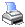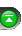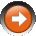﻿ Plot Class
 NinjaScript > Language Reference > Indicator > Plot ClassDefinition
Objects derived from the Plot class are used to characterize how a plot is visually displayed (plotted) on a chart.

Syntax

 Plot(Color color, string name) Plot(Pen pen, string name) Plot(Color color, PlotStyle plotStyle, string name) Plot(Pen pen, PlotStyle plotStyle, string name)

Properties

Plot.Min - Sets the minimum value required for the plot to display

Plot.Max - Sets the maximum value the plot will display

Please note that these properties are subject to a pixel rounding logic meaning that if your plot falls exactly on the .Min or .Max value, it is possible you would not see it painted depending on your panel height. To avoid this please set the .Min and .Max threshold a little bit wider than the minimum and maximum you desire.

* See tutorial "Custom Plot Color via Thresholds" for example of Plot.Min and Plot.Max properties.

Parameters

 color The line color (reference) name The plot name pen The pen used to draw the plot (reference) plotStyle The plot's PlotStyle:   PlotStyle.Bar PlotStyle.Block PlotStyle.Cross PlotStyle.Dot PlotStyle.Hash PlotStyle.HLine PlotStyle.Line PlotStyle.Square PlotStyle.TriangleDown PlotStyle.TriangleUp

Examples

See the Add() method for examples.